# 二进制、八进制、十进制和十六进制的相互转化（图解）

## 1. 二进制(B)、八进制(O)和十六进制(H) 转成十进制(D) 

• ### 二进制(B) --> 十进制(D)

方法(B -> D): 二进制数从低位到高位（即从右往左）计算，第0位的权值是2的0次方，第1位的权值是2的1次方，第2位的权值是2的2次方，依次递增下去，把最后的结果相加的值就是十进制的值了;

  二进制就是逢2进1，采用 0，1的组合来表达一个数;
// 例：将二进制的(101011)B转换为十进制的步骤如下;

第1位 1 x 2^0 = 1;

第2位 1 x 2^1 = 2;

第3位 0 x 2^2 = 0;

第4位 1 x 2^3 = 8;

第5位 0 x 2^4 = 0;

第6位 1 x 2^5 = 32;

读数: 把结果值相加，1+2+0+8+0+32=43，即(101011)B=(43)D

• ### 八进制(O) --> 十进制(D)

方法(O -> D): 八进制数从低位到高位（即从右往左）计算，第0位的权值是8的0次方，第1位的权值是8的1次方，第2位的权值是8的2次方，依次递增下去，把最后的结果相加的值就是十进制的值了;

  八进制就是逢8进1，八进制数采用 0～7这八数来表达一个数;
// 例：将八进制的(53)O转换为十进制的步骤如下;

第1位 3 x 8^0 = 3;

第2位 5 x 8^1 = 40;

读数: 把结果值相加，3+40=43，即(53)O=(43)D

• ### 十六进制(H) --> 十进制(D)

方法(H -> D): 十六进制数从低位到高位（即从右往左）计算，第0位的权值是16的0次方，第1位的权值是16的1次方，第2位的权值是16的2次方，依次递增下去，把最后的结果相加的值就是十进制的值了;

  十六进制就是逢16进1，十六进制的16个数为0, 1, 2, 3, 4, 5, 6, 7, 8, 9, A, B, C, D, E, F，其中A~F等值于十进制的10~15;
// 例：将十六进制的(2B)H转换为十进制的步骤如下;

第1位 B x 16^0 = 11;

第2位 2 x 16^1 = 32;

读数: 把结果值相加，11+32=43，即(2B)H=(43)D


## 2. 十进制(D) 转成二进制(B)、八进制(O)和十六进制(H)

• ### 十进制(D) --> 二进制(B)

方法(D -> B): 除2取余法，即每次将整数部分除以2，余数为该位权上的数，而商继续除以2，余数又为上一个位权上的数，这个步骤一直持续下去，直到商为0为止;

读数: 从最后一个余数读起，一直到最前面的一个余数;

  例：将十进制的(43)D转换为二进制的步骤如下;

1. 将商43除以2，商21余数为1;

2. 将商21除以2，商10余数为1;

3. 将商10除以2，商5余数为0;

4. 将商5除以2，商2余数为1;

5. 将商2除以2，商1余数为0;

6. 将商1除以2，商0余数为1;

读数: 因为最后一位是经过多次除以2才得到的，因此它是最高位，读数字从最后的余数向前读，101011，即(43)D=(101011)B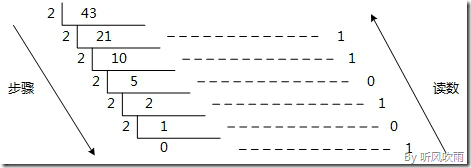• ### 十进制(D) --> 八进制(O)

方法1(D -> O): 除8取余法，即每次将整数部分除以8，余数为该位权上的数，而商继续除以8，余数又为上一个位权上的数，这个步骤一直持续下去，直到商为0为止;

读数: 从最后一个余数起，一直到最前面的一个余数;

  // 例：将十进制的(796)D转换为八进制的步骤如下;

1. 将商796除以8，商99余数为4;

2. 将商99除以8，商12余数为3;

4. 将商12除以8，商1余数为4;

5. 将商1除以8，商0余数为1;

读数: 因为最后一位是经过多次除以8才得到的，因此它是最高位，读数字从最后的余数向前读，1434，即(796)D=(1434)O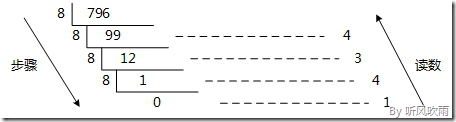方法2(D -> O): 使用间接法(D -> B -> O)，先将十进制转换成二进制，然后将二进制又转换成八进制;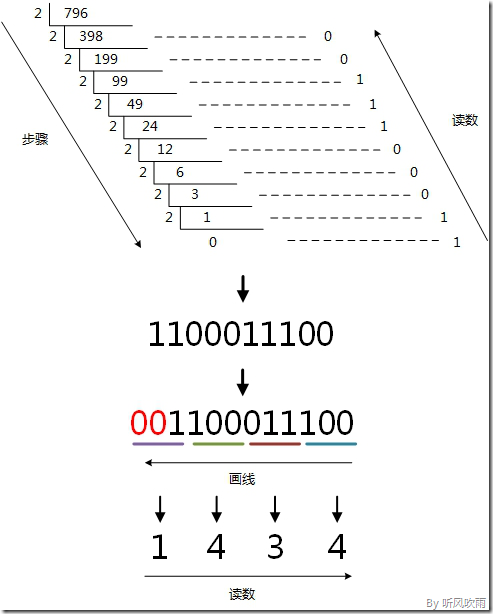• ### 十进制(D) --> 十六进制(H)

方法1(D -> H): 除16取余法，即每次将整数部分除以16，余数为该位权上的数，而商继续除以16，余数又为上一个位权上的数，这个步骤一直持续下去，直到商为0为止;

读数: 从最后一个余数起，一直到最前面的一个余数;

  // 例：将十进制的(796)D转换为十六进制的步骤如下;

1. 将商796除以16，商49余数为12，对应十六进制的C;

2. 将商49除以16，商3余数为1;

3. 将商3除以16，商0余数为3;

读数: 因为最后一位是经过多次除以16才得到的，因此它是最高位，读数字从最后的余数向前读，31C，即(796)D=(31C)H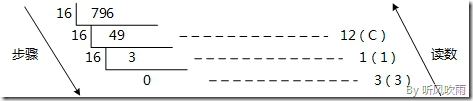方法2(D -> H): 使用间接法(D -> B -> H)，先将十进制转换成二进制，然后将二进制又转换成十六进制;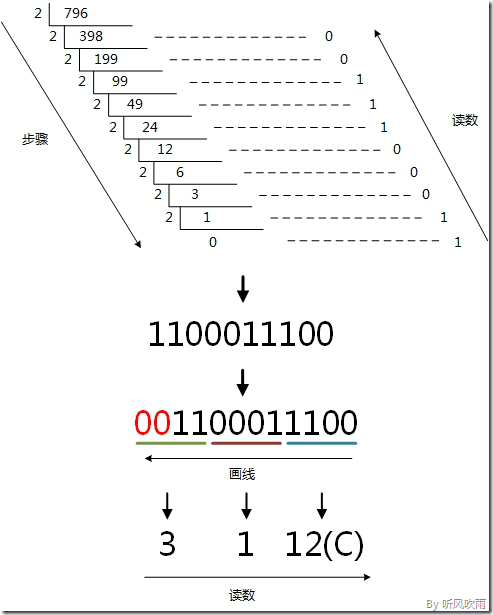## 3. 二进制(B)、八进制(O)和十六进制(H)互转

• ### 二进制(B) <--> 八进制(O)

方法(B -> O): 取三合一法，即从二进制的小数点为分界点，向左（向右）每三位取成一位，接着将这三位二进制按权拼接，然后按顺序进行排列，小数点的位置不变，得到的数字就是我们所求的八进制数;

如果向左（向右）取三位后，取到最高（最低）位时候，如果无法凑足三位，可以在小数点最左边（最右边），即整数的最高位（最低位）添0，凑足三位;

方法(O -> B): 取一分三法，即将一位八进制数分解成三位二进制数，用三位二进制按权相加去凑这位八进制数，小数点位置照旧;

  // 例：将二进制的(11010111.0100111)B转换为八进制的步骤如下(从小数点开始往两边画,不足三位补0);

1. 小数点前:

111 = 7;

010 = 2;

11补全为011, 011 = 3;

2. 小数点后:

010 = 2;

011 = 3;

1补全为100，100 = 4;

读数: 读数从高位到低位，即(11010111.0100111)B=(327.234)O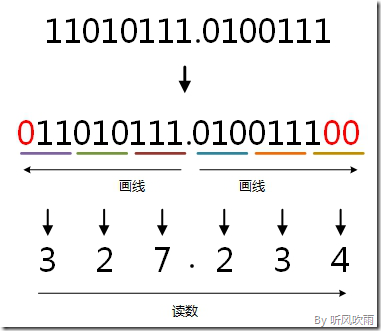二进制和八进制编码对应表

二进制(B) 八进制(O)
000 0
001 1
010 2
011 3
100 4
101 5
110 6
111 7
• ### 二进制(B) <--> 十六进制(H)

方法(B -> H): 取四合一法，即从二进制的小数点为分界点，向左（向右）每四位取成一位，接着将这四位二进制按权相拼接，然后按顺序进行排列，小数点的位置不变，得到的数字就是我们所求的十六进制数;

如果向左（向右）取四位后，取到最高（最低）位时候，如果无法凑足四位，可以在小数点最左边（最右边），即整数的最高位（最低位）添0，凑足四位;

方法(H -> B): 取一分四法，即将一位十六进制数分解成四位二进制数，用四位二进制按权相加去凑这位十六进制数，小数点位置照旧;

  // 例：将二进制的(11010111)B转换为十六进制的步骤如下(从右往左画,不足四位补0)：

0111 = 7;

1101 = D;

读数: 读数从高位到低位，即(11010111)B=(D7)H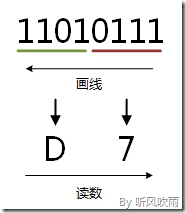二进制和十六进制编码对应表

二进制(B) 十六进制(O)
0000 0
0001 1
0010 2
0011 3
0100 4
0101 5
0110 6
0111 7
1000 8
1001 9
1010 A
1011 B
1100 C
1101 D
1110 E
1111 F
• ### 八进制(O) <--> 十六进制(H)

方法(O -> H): 使用间接法(O -> B -> H)，先将八进制转换为二进制，然后再将二进制转换为十六进制，小数点位置不变;

方法(H -> O): 使用间接法(H -> B -> O)，先将十六进制转换为二进制，然后再将二进制转换为八进制，小数点位置不变;

  // 例：将八进制的(327)O转换为十六进制的步骤如下：

1. 八进制(327)O转换成二进制;

3 = 011;

2 = 010;

7 = 111;
2. 得到(011010111)B, 再转换成十六进制;

0111 = 7;

1101 = D;

读数: 读数从高位到低位，D7，即(327)O=(D7)H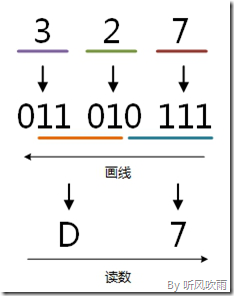## 4. 扩展阅读

• 幂计算

  // 1. 包含小数的进制换算：

(ABC.8C)H = 10x16^2 + 11x16^1 + 12x16^0 + 8x16^-1 + 12x16^-2

= 10x16^2 + 11x16^1 + 12x16^0 + 8x(1/16^1) + 12x(1/16^2)

= 2560 + 176 + 12 + 0.5 + 0.046875

= (2748.546875)D

// 2. 负次幂的计算：

2^-5 = 2^(0-5)

= 2^0 / 2^5

= 1 / 2^5


1. 在数字后面加上不同的字母来表示不同的进位制。B（Binary)表示二进制，O（Octal）表示八进制，D（Decimal）或不加表示十进制，H（Hexadecimal）表示十六进制。例如：(101011)B = (53)O = (43)D = (2B)H ↩︎

posted @ 2021-05-18 12:06  Librarookie  阅读(517)  评论(0编辑  收藏  举报